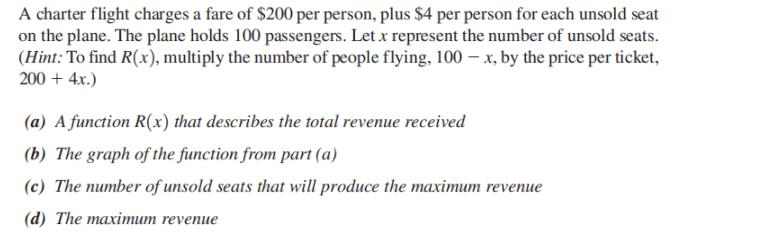### ¿Todavía tienes preguntas de matemáticas?

Pregunte a nuestros tutores expertos
Algebra
PreguntaA charter flight charges a fare of $$\ 200$$ per person, plus $$\ 4$$ per person for each unsold seat on the plane. The plane holds $$100$$ passengers. Let $$x$$ represent the number of unsold seats. (Hint: To find $$R ( x )$$ , multiply the number of people flying, $$100 - x$$ , by the price per ticket, $$200 + 4 x$$ .)

(a) A function $$R ( x )$$ that describes the total revenue received

(b) The graph of the function from part (a)

(c) The number of unsold seats that will produce the maximum revenue

(d) The maximum revenue

$$\left. \begin{array} { l }{ \text { (a) } R ( x ) = ( 100 - x ) ( 200 + 4 x ) }\\{ = 20,000 + 200 x - 4 x ^ { 2 } }\\{ \text { (b) } \text { (c) } 25 \quad \text { (d) } \ 22,500 } \end{array} \right.$$